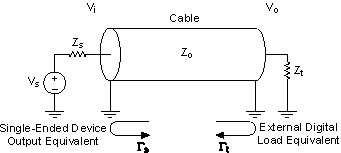# Transmission Lines

NI Digital Waveform Generator/Analyzer Help

Edition Date: June 2013

Part Number: 370520P-01

»View Product InfoDownload Help (Windows Only)

In lower frequency (slow edge rate) applications, you can assume that small wires interconnecting devices do not affect system performance and that every point in a wire has the same voltage as every other point for any instance in time. At lower frequencies, this "lumped" circuit model is valid. However, as frequency content increases, even the small geometries of typical wire dimensions become a significant portion of the signal wavelengths, in which case the small inductance and capacitance become electrically significant impedances.

What constitutes a significant proportion of the wavelength? While this value changes for different applications, for digital circuits, a good general rule is that if the propagation delay in a wire or interconnect is greater than one-sixth of the rise time of the digital signal, then the "lumped" circuit analysis assumption is no longer valid and you should analyze the interconnect as a transmission line.

For calculation purposes, you should understand the concept of electrical length (l). Electrical length is defined as the distance that a signal can travel in an electrical medium during the time that it takes for one rise or fall time, whichever is longer. Using the concept of electrical length, the general rule of the previous paragraph can be rephrased as follows: If the physical length of a wire or electrical interconnect is greater than one-sixth of the electrical length of a signal propagating on that wire, the system must be analyzed as a transmission line.

Velocity is defined as the rate at which an electrical wave propagates in the transmission medium. Using this value you can calculate electrical length in one of the following ways:

• l(in) = Velocity(in/ns) • trise
• l(in) = trise(ns)/tpd(ns/in)

where trise is the rise/fall time of the digital edge, and tpd is the propagation delay of the edge in the transmission line.

For example, the NI SHC68-C68-D2 shielded cable has a tpd of 165 ps/inch. On an NI 6551/6552 device, trise for a high-speed digital signal can be as low as 1.5 ns. Therefore, the electrical length is 9 in. (trise/tpd =  1.5/.165 = 9 in.), and any trace lengths longer than 1.5 in. (9 in./6) should be treated as a transmission line. This cable is significantly longer than 1.5 in., so NI considers this cable to be a transmission line and designed the cable to have 50 Ω characteristic impedance.Note  While the propagation delay number in the previous example is specific to the NI SHC68-C68-D2 cable, if you do not know the specific propagation delays for your interconnects, when using the NI digital waveform generator/analyzer, assume that you are working with transmission lines for any wire or interconnect longer than 1 to 2 inches.

The following figure gives a simple diagram of a basic single-ended transmission line. A voltage source (Vs) generates a digital edge with an impedance of Zs looking "into" the transmission line. The transmission line itself has some low characteristic AC impedance (Z0) to ground, typically 50 Ω for most test systems. The end of the transmission line is most commonly terminated through an impedance (Zt) to ground at the destination.Practically, termination at only one end of the transmission line is often adequate and is more commonly used. However, for high-precision applications, termination at both the source and the load end of the transmission line yields the best results.